# Calculus 3 : Divergence

## Example Questions

1 2 3 4 5 7 Next →

### Example Question #67 : Line Integrals

Find the divergence of the vector field: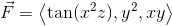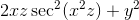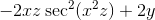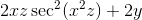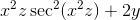Explanation:

The divergence of a vector field is given by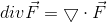, where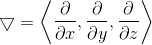When we take the dot product of the gradient and the vector field, we get the sum of the respective partial derivatives. To find the given partial derivative of the function, we must treat the other variable(s) as constants.

The partial derivatives are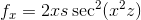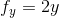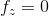### Example Question #68 : Line Integrals

Find the divergence of the vector field: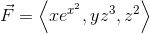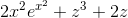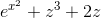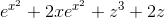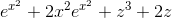Explanation:

The divergence of a vector field is given by, whereWhen we take the dot product of the gradient and the vector field, we get the sum of the respective partial derivatives. To find the given partial derivative of the function, we must treat the other variable(s) as constants.

The partial derivatives are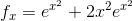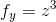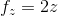### Example Question #69 : Line Integrals

Find the divergence of the vector field: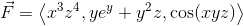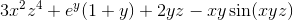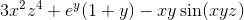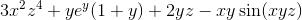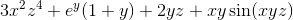Explanation:

The divergence of a vector field is given by, whereIn taking the dot product of the gradient with the vector field, we get the sum of the respective partial derivatives. To find the given partial derivative of the function, we must treat the other variable(s) as constants.

The partial derivatives are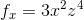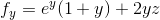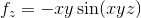### Example Question #70 : Line Integrals

Find the divergence of the vector field: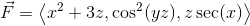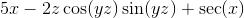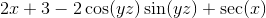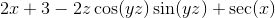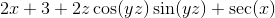Explanation:

The divergence of a vector field is given by, where.

When we take the dot product of the gradient and the vector field, we get the sum of the respective partial derivatives. To find the given partial derivative of the function, we must treat the other variable(s) as constants.

The partial derivatives are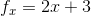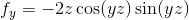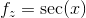### Example Question #71 : Line Integrals

Find the divergence of the vector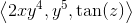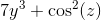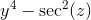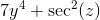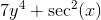Explanation:

To find the divergence of a vector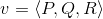, we use the formula: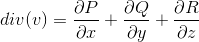Applying to the vector from the problem statement, we get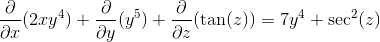### Example Question #72 : Line Integrals

Find the divergence of the vector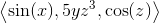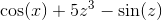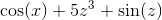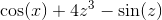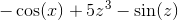Explanation:

To find the divergence of a vector, we use the formula:Applying to the vector from the problem statement, we get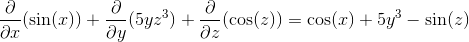### Example Question #73 : Line Integrals

Find the divergence of the function: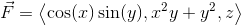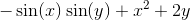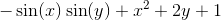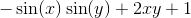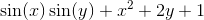Explanation:

The divergence of a vector field is given by, whereWhen we take the dot product of the gradient and the vector field, we get the sum of the respective partial derivatives. To find the given partial derivative of the function, we must treat the other variable(s) as constants.

The partial derivatives are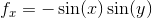,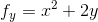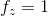### Example Question #74 : Line Integrals

Find the divergence of the vector field: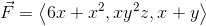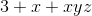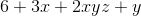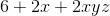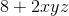Explanation:

The divergence of a vector field is given by, whereWhen we take the dot product of the gradient and the vector field, we get the sum of the respective partial derivatives. To find the given partial derivative of the function, we must treat the other variable(s) as constants.

The partial derivatives are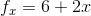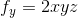### Example Question #75 : Line Integrals

Find the divergence of the vector field: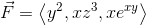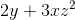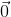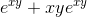Explanation:

The divergence of a vector field is given by, whereWhen we take the dot product of the gradient and the vector field, we get the sum of the respective partial derivatives. To find the given partial derivative of the function, we must treat the other variable(s) as constants.

The partial derivatives are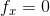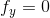1 2 3 4 5 7 Next →